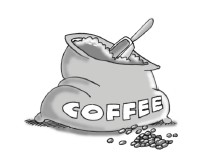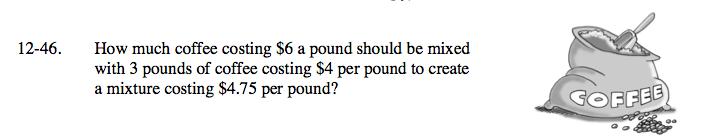### Home > AC > Chapter 12 > Lesson 12.2.2 > Problem12-46

12-46.How much coffee costing $6 a pound should be mixed with 3 pounds of coffee costing$4 per pound to create a mixture costing $4.75 per pound? Homework Help ✎Define your variables. x = # of pounds of$6 coffee

Write an equation to represent the problem. Use formula
(cost per pound)(pounds) = (cost per pound)(pounds)

6x + 12 = 4.75(x + 3)

Solve for x.